Скачать презентацию EECS 110 Lec 11 Indefinite Loops and Program

4143f71dee2f0ac09b978887135c38cb.ppt

• Количество слайдов: 54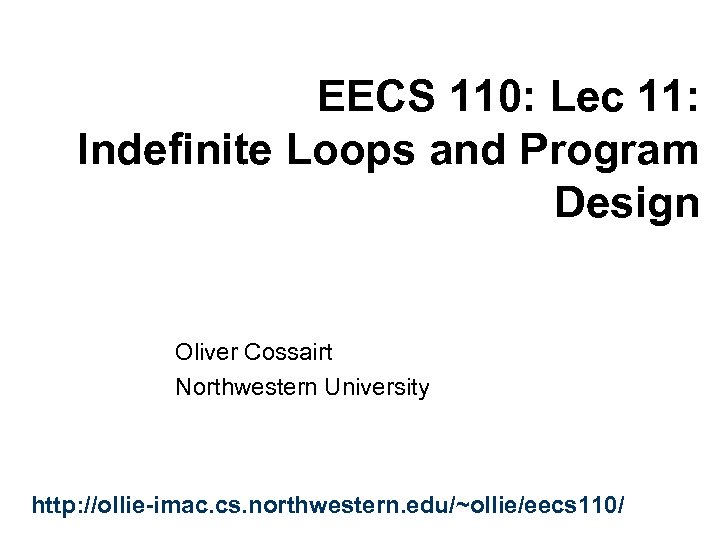EECS 110: Lec 11: Indefinite Loops and Program Design Oliver Cossairt Northwestern University http: //ollie-imac. cs. northwestern. edu/~ollie/eecs 110/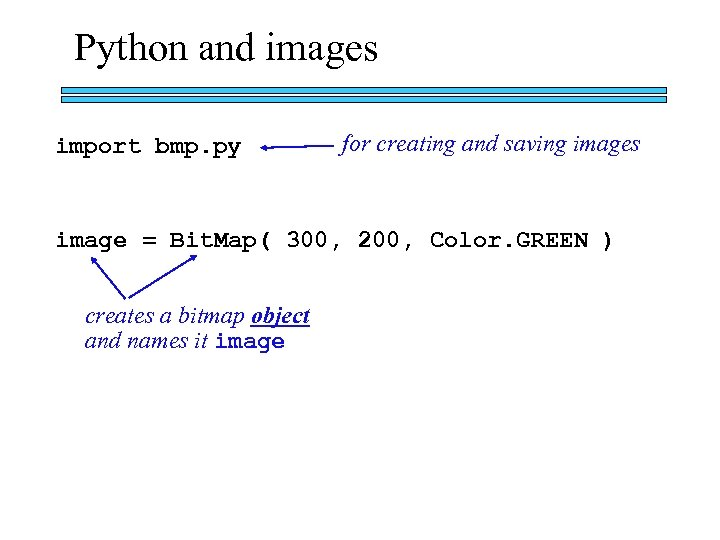Python and images import bmp. py for creating and saving images image = Bit. Map( 300, 200, Color. GREEN ) creates a bitmap object and names it image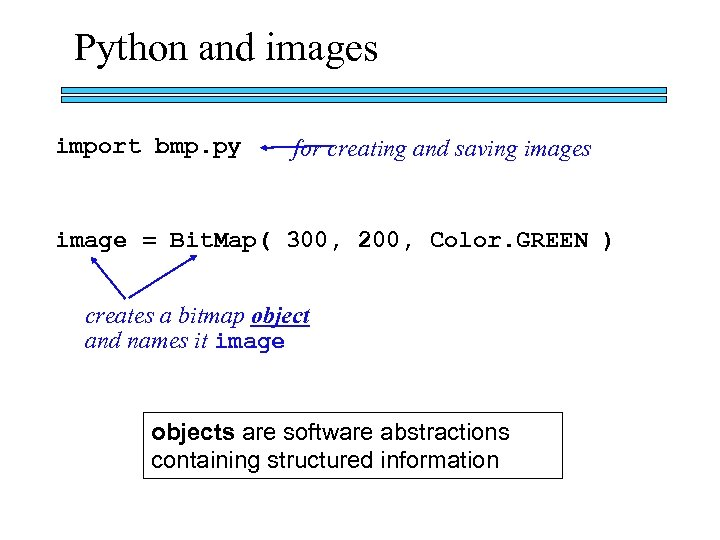Python and images import bmp. py for creating and saving images image = Bit. Map( 300, 200, Color. GREEN ) creates a bitmap object and names it image objects are software abstractions containing structured information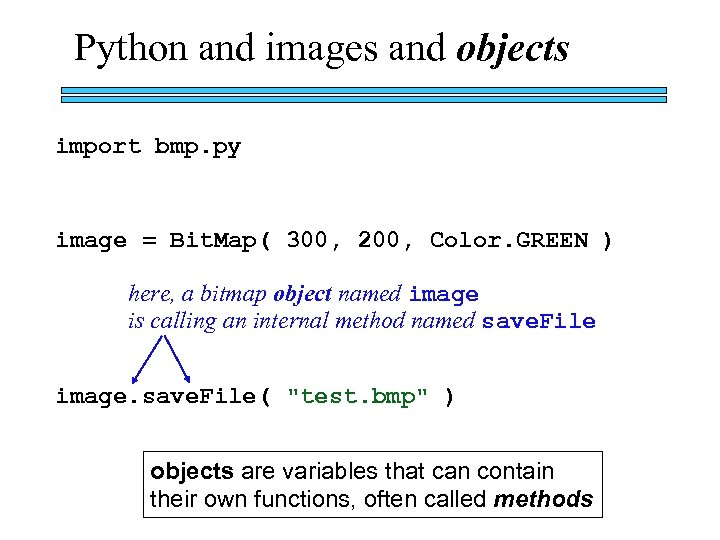Python and images and objects import bmp. py image = Bit. Map( 300, 200, Color. GREEN ) here, a bitmap object named image is calling an internal method named save. File image. save. File( "test. bmp" ) objects are variables that can contain their own functions, often called methods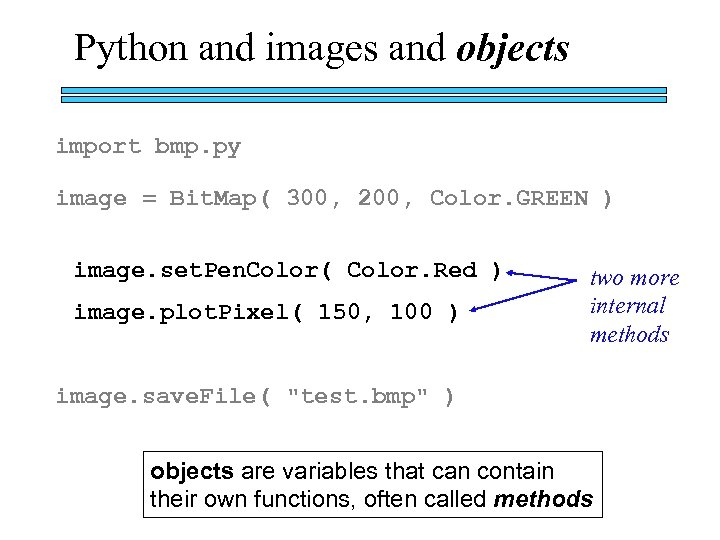Python and images and objects import bmp. py image = Bit. Map( 300, 200, Color. GREEN ) image. set. Pen. Color( Color. Red ) image. plot. Pixel( 150, 100 ) two more internal methods image. save. File( "test. bmp" ) objects are variables that can contain their own functions, often called methods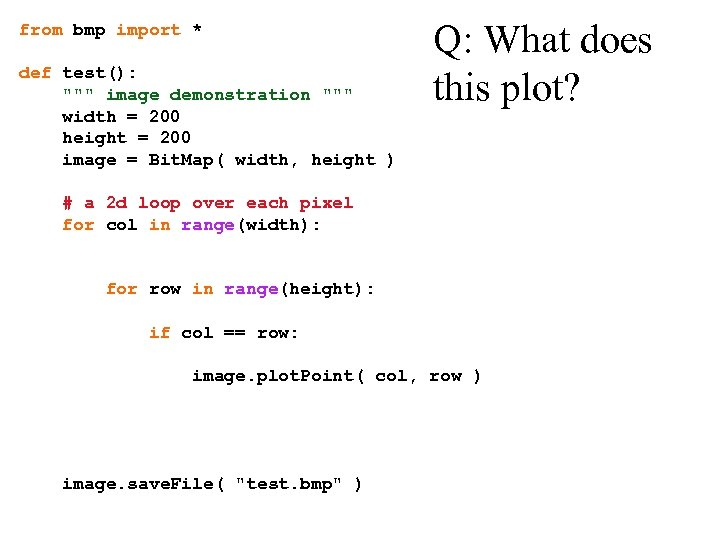from bmp import * def test(): """ image demonstration """ width = 200 height = 200 image = Bit. Map( width, height ) Q: What does this plot? # a 2 d loop over each pixel for col in range(width): for row in range(height): if col == row: image. plot. Point( col, row ) image. save. File( "test. bmp" )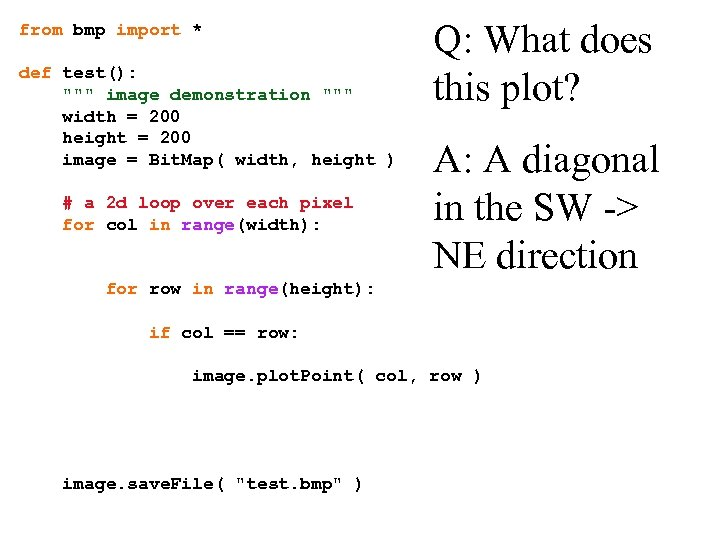from bmp import * def test(): """ image demonstration """ width = 200 height = 200 image = Bit. Map( width, height ) # a 2 d loop over each pixel for col in range(width): Q: What does this plot? A: A diagonal in the SW -> NE direction for row in range(height): if col == row: image. plot. Point( col, row ) image. save. File( "test. bmp" )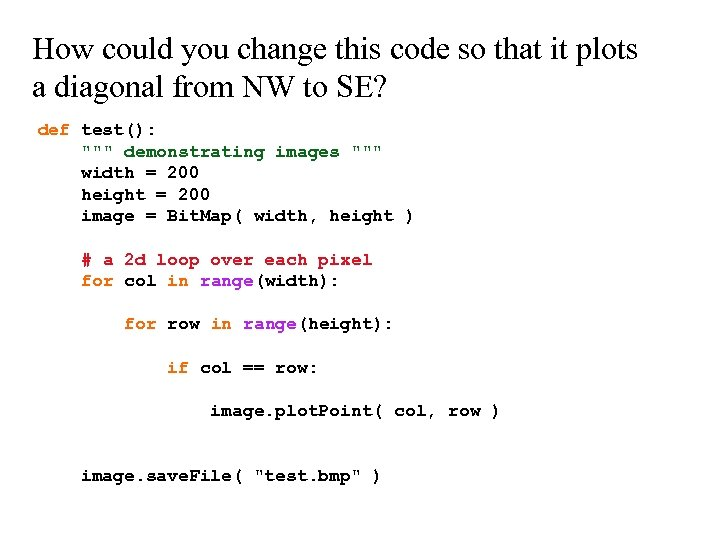How could you change this code so that it plots a diagonal from NW to SE? def test(): """ demonstrating images """ width = 200 height = 200 image = Bit. Map( width, height ) # a 2 d loop over each pixel for col in range(width): for row in range(height): if col == row: image. plot. Point( col, row ) image. save. File( "test. bmp" )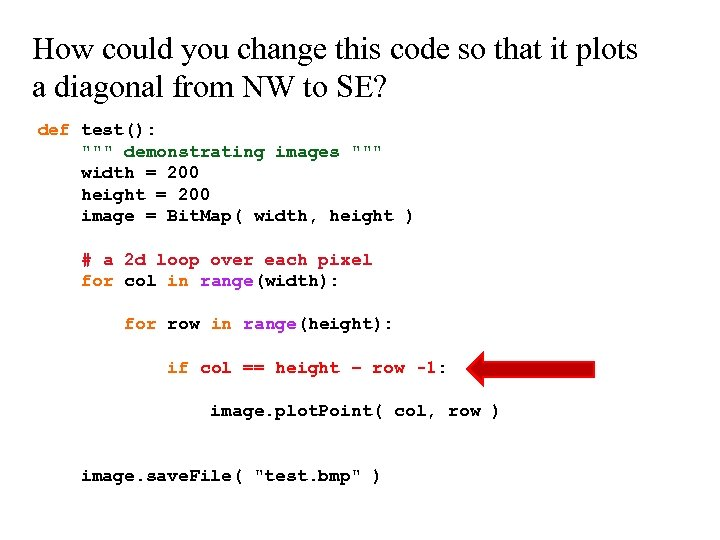How could you change this code so that it plots a diagonal from NW to SE? def test(): """ demonstrating images """ width = 200 height = 200 image = Bit. Map( width, height ) # a 2 d loop over each pixel for col in range(width): for row in range(height): if col == height – row -1: image. plot. Point( col, row ) image. save. File( "test. bmp" )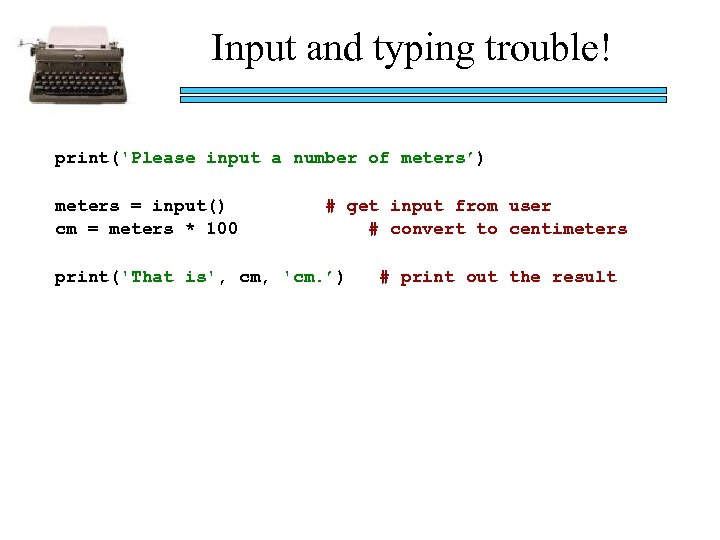Input and typing trouble! print('Please input a number of meters’) meters = input() cm = meters * 100 # get input from user # convert to centimeters print('That is', cm, 'cm. ’) # print out the result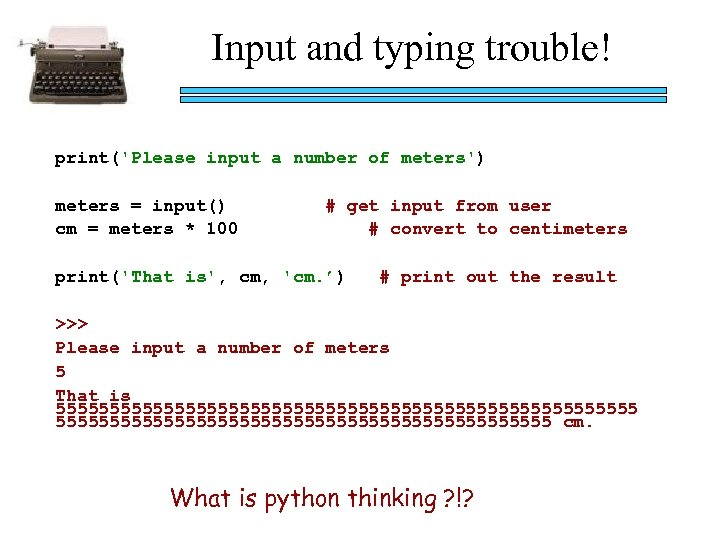Input and typing trouble! print('Please input a number of meters') meters = input() cm = meters * 100 # get input from user # convert to centimeters print('That is', cm, 'cm. ’) # print out the result >>> Please input a number of meters 5 That is 555555555555555555555555555 cm. What is python thinking ? !?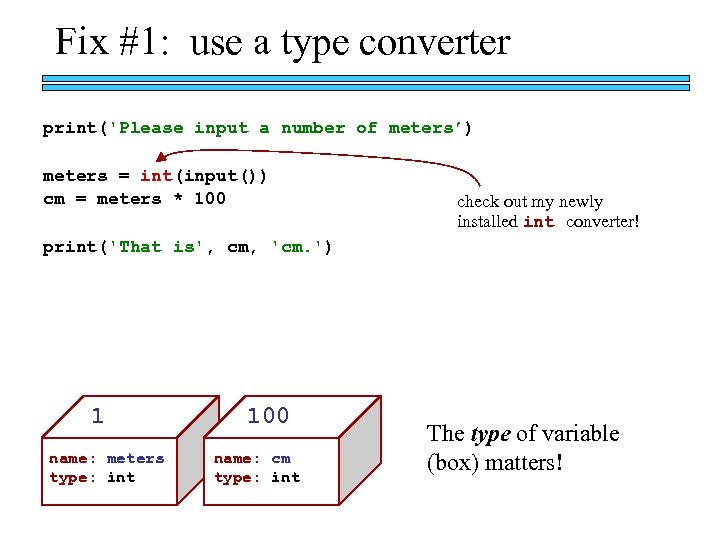Fix #1: use a type converter print('Please input a number of meters’) meters = int(input()) cm = meters * 100 check out my newly installed int converter! print('That is', cm, 'cm. ') 1 name: meters type: int 100 name: cm type: int The type of variable (box) matters!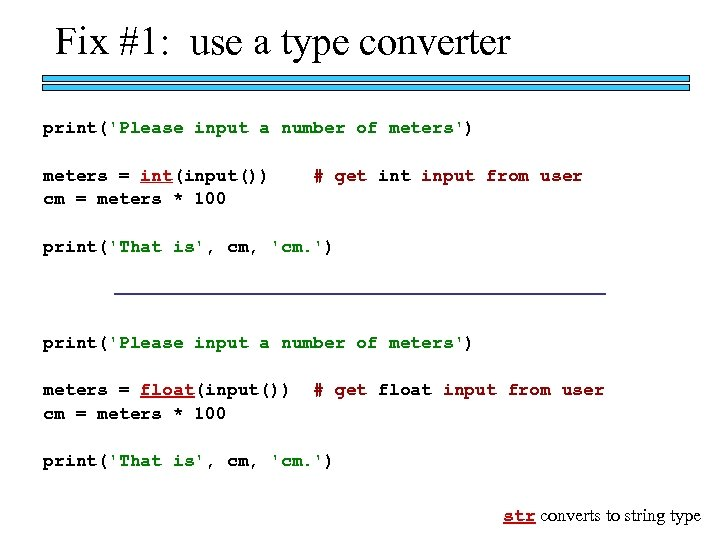Fix #1: use a type converter print('Please input a number of meters') meters = int(input()) cm = meters * 100 # get input from user print('That is', cm, 'cm. ') print('Please input a number of meters') meters = float(input()) cm = meters * 100 # get float input from user print('That is', cm, 'cm. ') str converts to string type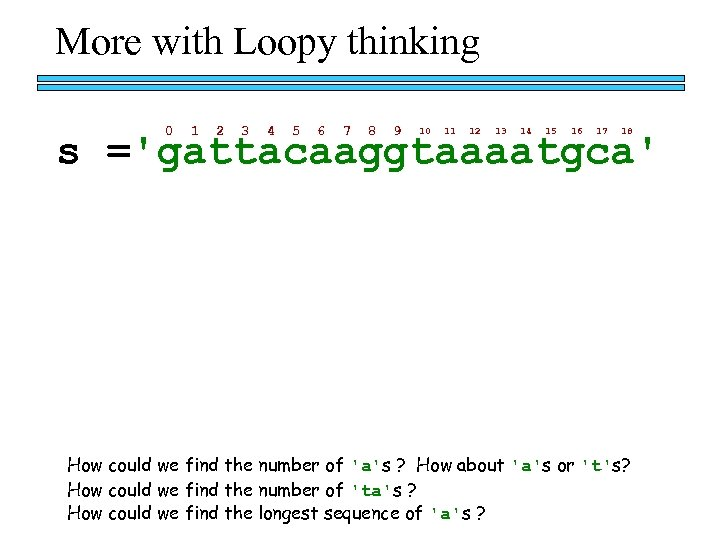More with Loopy thinking 0 1 2 3 4 5 6 7 8 9 10 11 12 13 14 15 16 17 18 s ='gattacaaggtaaaatgca' How could we find the number of 'a's ? How about 'a's or 't's? How could we find the number of 'ta's ? How could we find the longest sequence of 'a's ?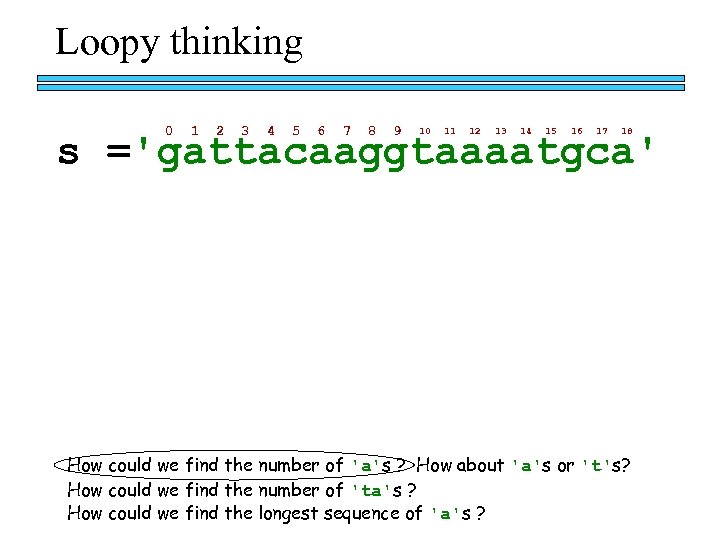Loopy thinking 0 1 2 3 4 5 6 7 8 9 10 11 12 13 14 15 16 17 18 s ='gattacaaggtaaaatgca' How could we find the number of 'a's ? How about 'a's or 't's? How could we find the number of 'ta's ? How could we find the longest sequence of 'a's ?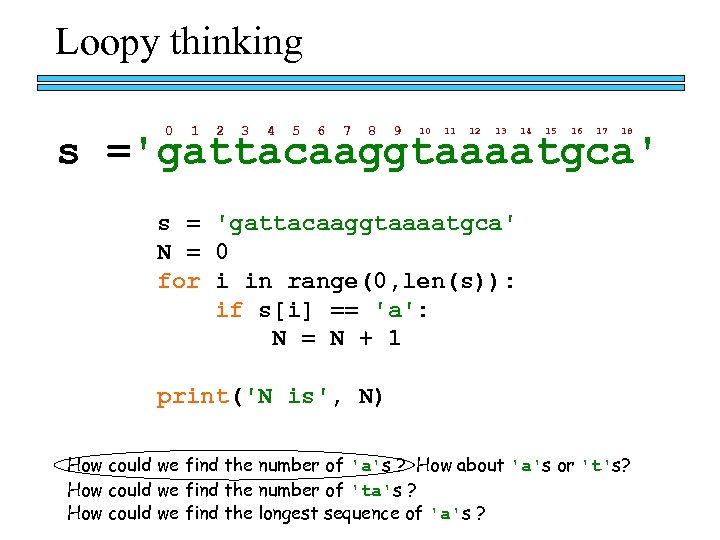Loopy thinking 0 1 2 3 4 5 6 7 8 9 10 11 12 13 14 15 16 17 18 s ='gattacaaggtaaaatgca' s = 'gattacaaggtaaaatgca' N = 0 for i in range(0, len(s)): if s[i] == 'a': N = N + 1 print('N is', N) How could we find the number of 'a's ? How about 'a's or 't's? How could we find the number of 'ta's ? How could we find the longest sequence of 'a's ?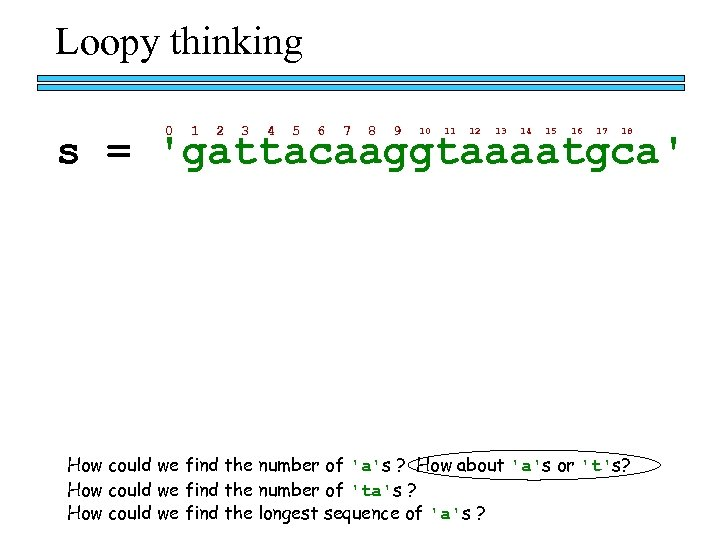Loopy thinking 0 1 2 3 4 5 6 7 8 9 10 11 12 13 14 15 16 17 18 s = 'gattacaaggtaaaatgca' How could we find the number of 'a's ? How about 'a's or 't's? How could we find the number of 'ta's ? How could we find the longest sequence of 'a's ?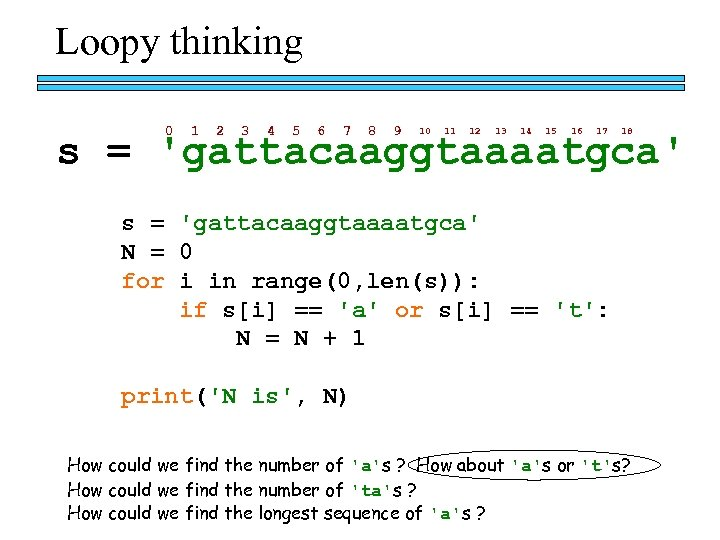Loopy thinking 0 1 2 3 4 5 6 7 8 9 10 11 12 13 14 15 16 17 18 s = 'gattacaaggtaaaatgca' N = 0 for i in range(0, len(s)): if s[i] == 'a' or s[i] == 't': N = N + 1 print('N is', N) How could we find the number of 'a's ? How about 'a's or 't's? How could we find the number of 'ta's ? How could we find the longest sequence of 'a's ?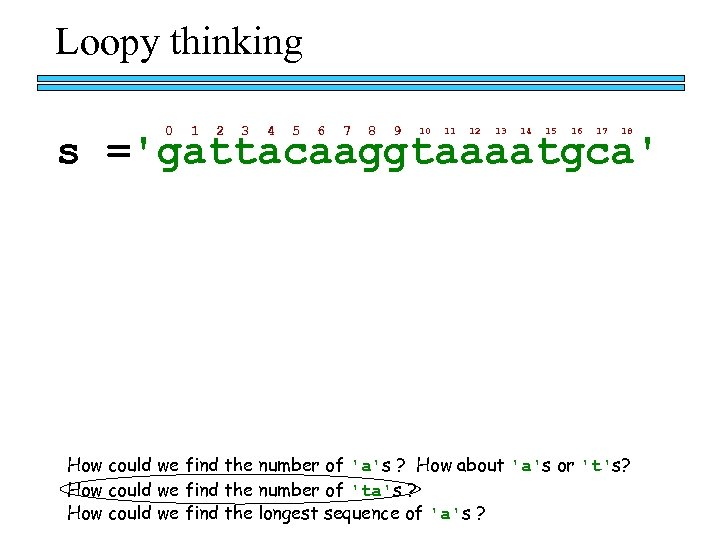Loopy thinking 0 1 2 3 4 5 6 7 8 9 10 11 12 13 14 15 16 17 18 s ='gattacaaggtaaaatgca' How could we find the number of 'a's ? How about 'a's or 't's? How could we find the number of 'ta's ? How could we find the longest sequence of 'a's ?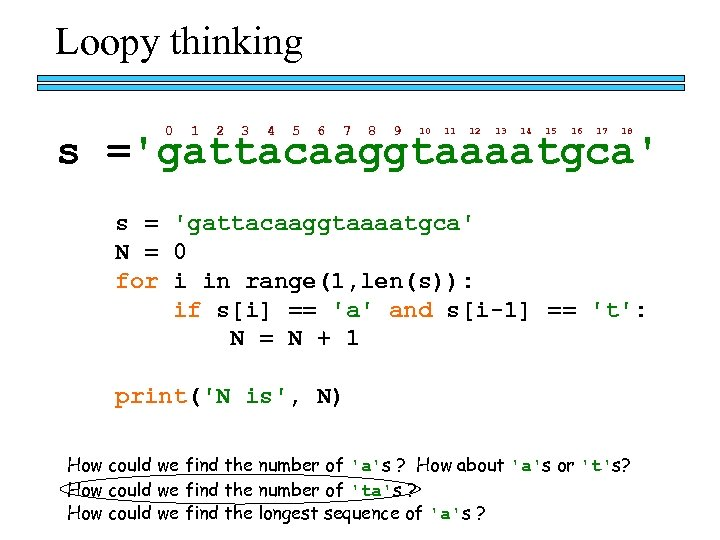Loopy thinking 0 1 2 3 4 5 6 7 8 9 10 11 12 13 14 15 16 17 18 s ='gattacaaggtaaaatgca' s = 'gattacaaggtaaaatgca' N = 0 for i in range(1, len(s)): if s[i] == 'a' and s[i-1] == 't': N = N + 1 print('N is', N) How could we find the number of 'a's ? How about 'a's or 't's? How could we find the number of 'ta's ? How could we find the longest sequence of 'a's ?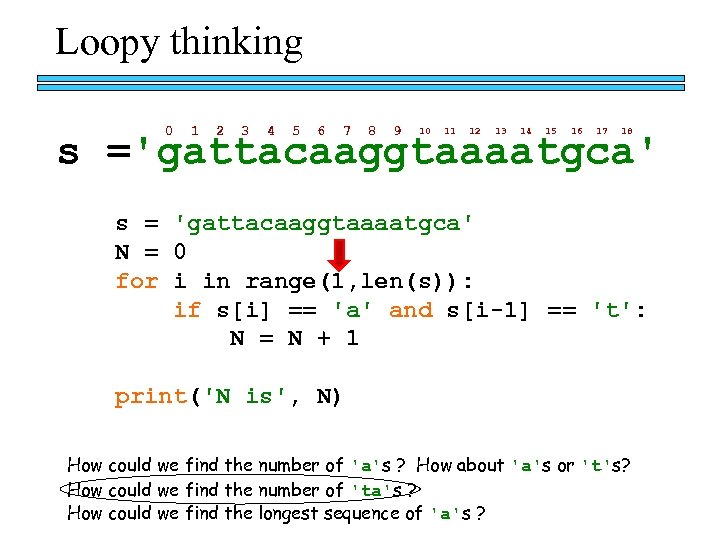Loopy thinking 0 1 2 3 4 5 6 7 8 9 10 11 12 13 14 15 16 17 18 s ='gattacaaggtaaaatgca' s = 'gattacaaggtaaaatgca' N = 0 for i in range(1, len(s)): if s[i] == 'a' and s[i-1] == 't': N = N + 1 print('N is', N) How could we find the number of 'a's ? How about 'a's or 't's? How could we find the number of 'ta's ? How could we find the longest sequence of 'a's ?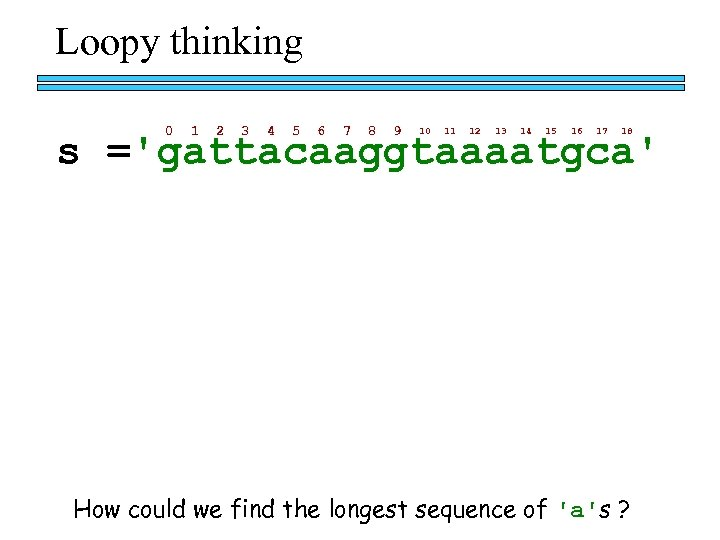Loopy thinking 0 1 2 3 4 5 6 7 8 9 10 11 12 13 14 15 16 17 18 s ='gattacaaggtaaaatgca' How could we find the longest sequence of 'a's ?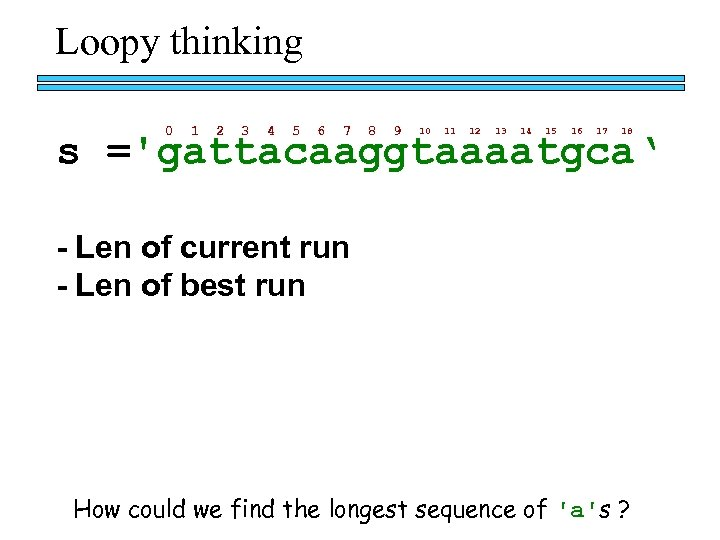Loopy thinking 0 1 2 3 4 5 6 7 8 9 10 11 12 13 14 15 16 17 18 s ='gattacaaggtaaaatgca‘ - Len of current run - Len of best run How could we find the longest sequence of 'a's ?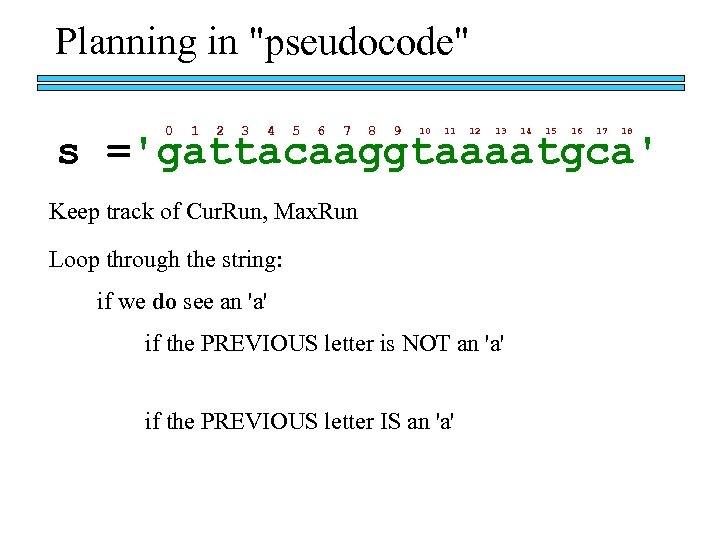Planning in "pseudocode" 0 1 2 3 4 5 6 7 8 9 10 11 12 13 14 15 16 17 18 s ='gattacaaggtaaaatgca' Keep track of Cur. Run, Max. Run Loop through the string: if we do see an 'a' if the PREVIOUS letter is NOT an 'a' if the PREVIOUS letter IS an 'a'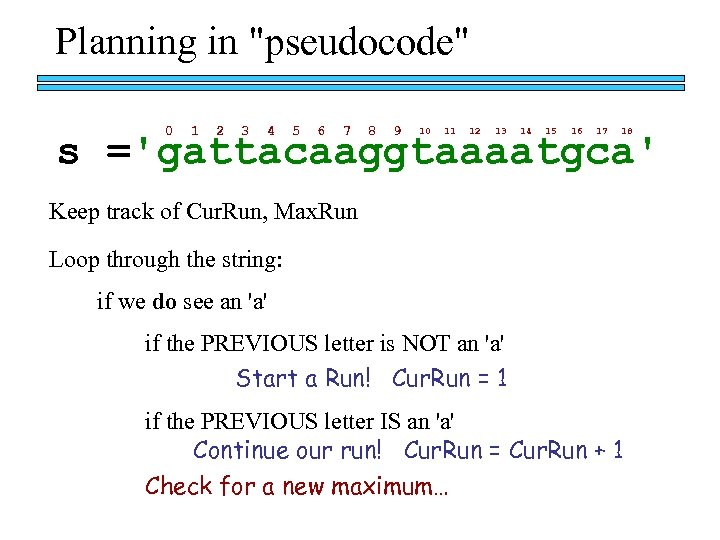Planning in "pseudocode" 0 1 2 3 4 5 6 7 8 9 10 11 12 13 14 15 16 17 18 s ='gattacaaggtaaaatgca' Keep track of Cur. Run, Max. Run Loop through the string: if we do see an 'a' if the PREVIOUS letter is NOT an 'a' Start a Run! Cur. Run = 1 if the PREVIOUS letter IS an 'a' Continue our run! Cur. Run = Cur. Run + 1 Check for a new maximum…Planning in "pseudocode" 0 1 2 3 4 5 6 7 8 9 10 11 12 13 14 15 16 17 18 s ='gattacaaggtaaaatgca' MAX = 0 cur = 0 Loop through the string: for i in range(0, len(s)): if we do see an 'a' if s[i] == 'a': if the PREVIOUS letter is NOT an 'a' if s[i-1] != 'a': Start a Run! cur = 1 if the PREVIOUS letter IS an 'a' else: Continue our run! cur = cur + 1 if cur > MAX: Check for a new maximum… MAX = cur Keep track of Cur. Run, Max. Run print('Max is', MAX)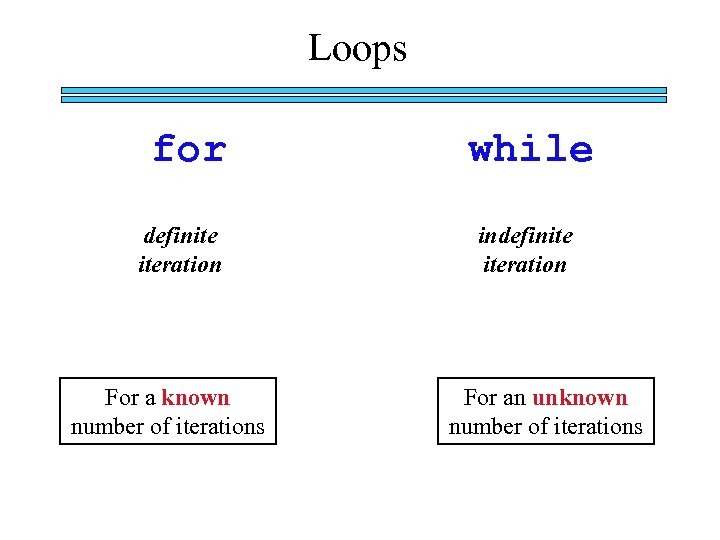Loops for definite iteration For a known number of iterations while indefinite iteration For an unknown number of iterations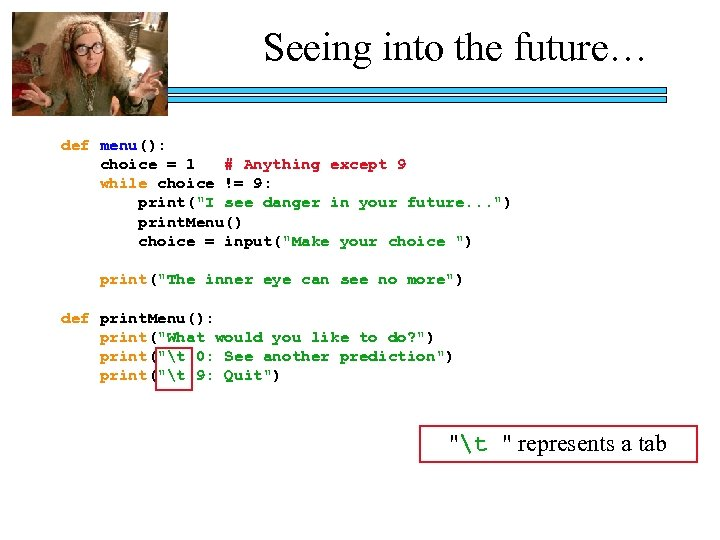Seeing into the future… def menu(): choice = 1 # Anything except 9 while choice != 9: print("I see danger in your future. . . ") print. Menu() choice = input("Make your choice ") print("The inner eye can see no more") def print. Menu(): print("What would you like to do? ") print("t 0: See another prediction") print("t 9: Quit") "t " represents a tab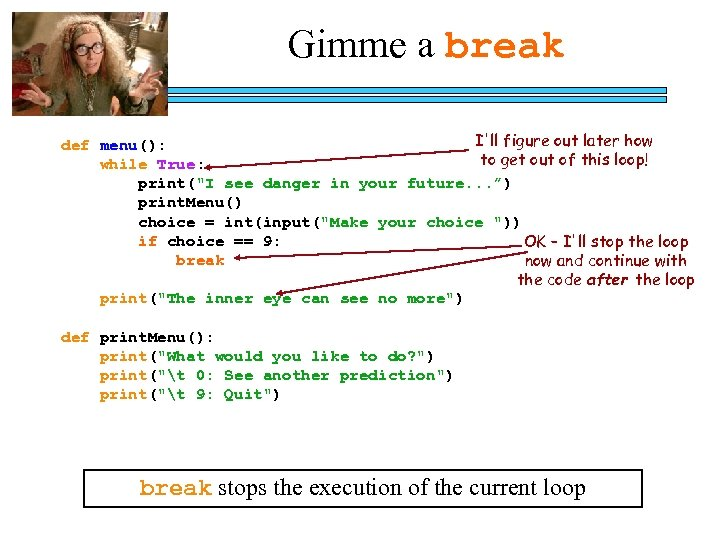Gimme a break I'll figure out later how def menu(): to get out of this loop! while True: print("I see danger in your future. . . ”) print. Menu() choice = int(input("Make your choice ")) if choice == 9: OK – I'll stop the loop break now and continue with the code after the loop print("The inner eye can see no more") def print. Menu(): print("What would you like to do? ") print("t 0: See another prediction") print("t 9: Quit") break stops the execution of the current loop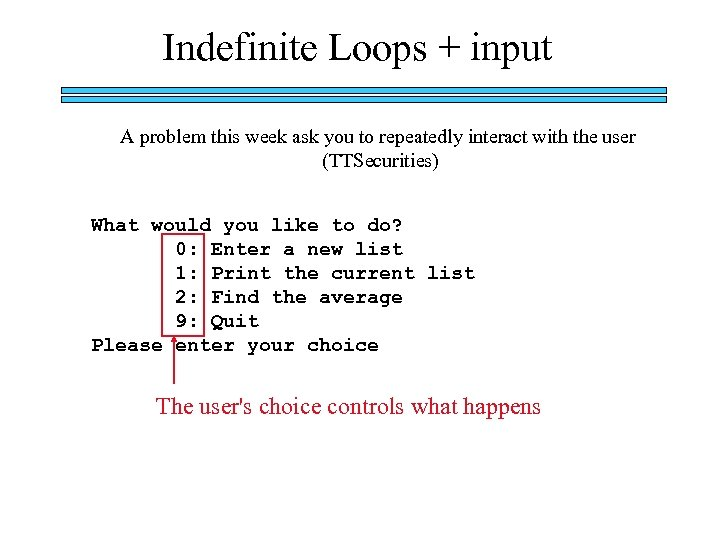Indefinite Loops + input A problem this week ask you to repeatedly interact with the user (TTSecurities) What would you like to do? 0: Enter a new list 1: Print the current list 2: Find the average 9: Quit Please enter your choice The user's choice controls what happens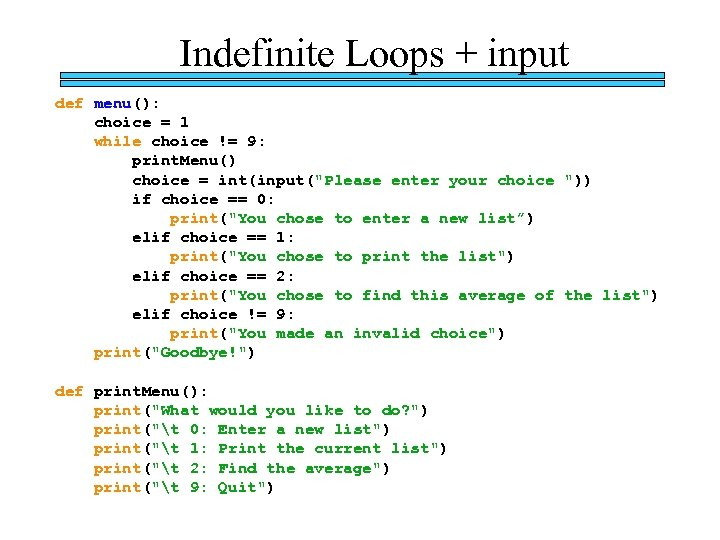Indefinite Loops + input def menu(): choice = 1 while choice != 9: print. Menu() choice = int(input("Please enter your choice ")) if choice == 0: print("You chose to enter a new list”) elif choice == 1: print("You chose to print the list") elif choice == 2: print("You chose to find this average of the list") elif choice != 9: print("You made an invalid choice") print("Goodbye!") def print. Menu(): print("What would you like to do? ") print("t 0: Enter a new list") print("t 1: Print the current list") print("t 2: Find the average") print("t 9: Quit")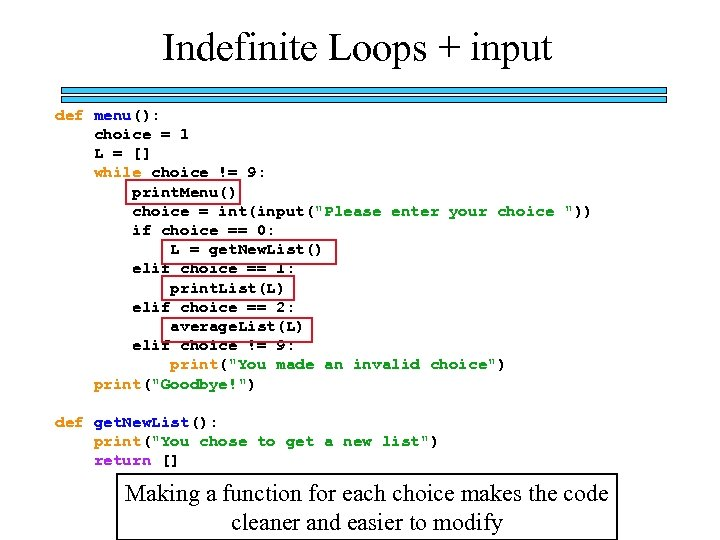Indefinite Loops + input def menu(): choice = 1 L = [] while choice != 9: print. Menu() choice = int(input("Please enter your choice ")) if choice == 0: L = get. New. List() elif choice == 1: print. List(L) elif choice == 2: average. List(L) elif choice != 9: print("You made an invalid choice") print("Goodbye!") def get. New. List(): print("You chose to get a new list") return [] Making a function for each choice makes the code cleaner and easier to modify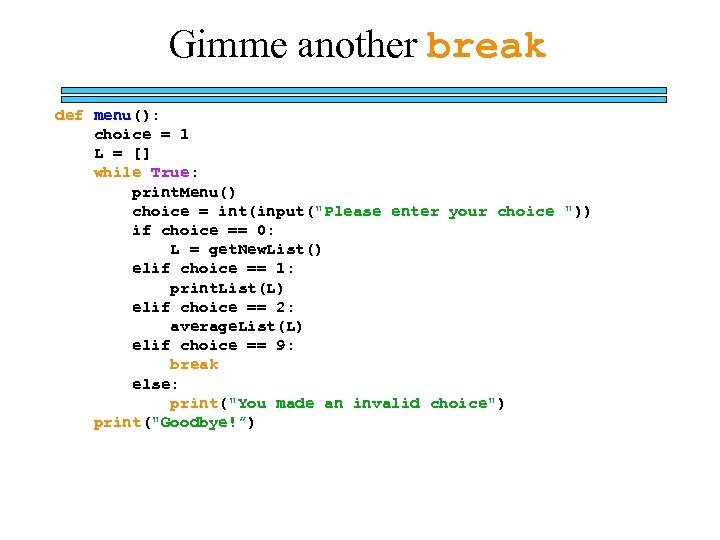Gimme another break def menu(): choice = 1 L = [] while True: print. Menu() choice = int(input("Please enter your choice ")) if choice == 0: L = get. New. List() elif choice == 1: print. List(L) elif choice == 2: average. List(L) elif choice == 9: break else: print("You made an invalid choice") print("Goodbye!”)The Price Is Right! Goal: Buy from a set of 5 items (as many of each as you want) while spending between \$9. 25 and \$10. 00.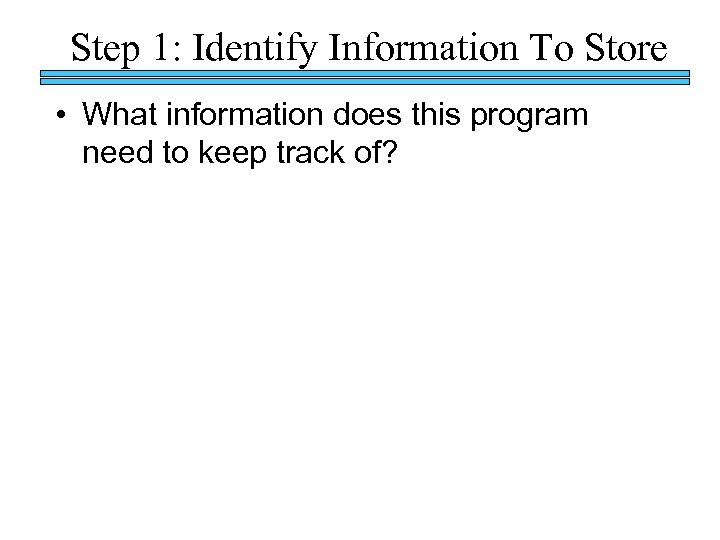Step 1: Identify Information To Store • What information does this program need to keep track of?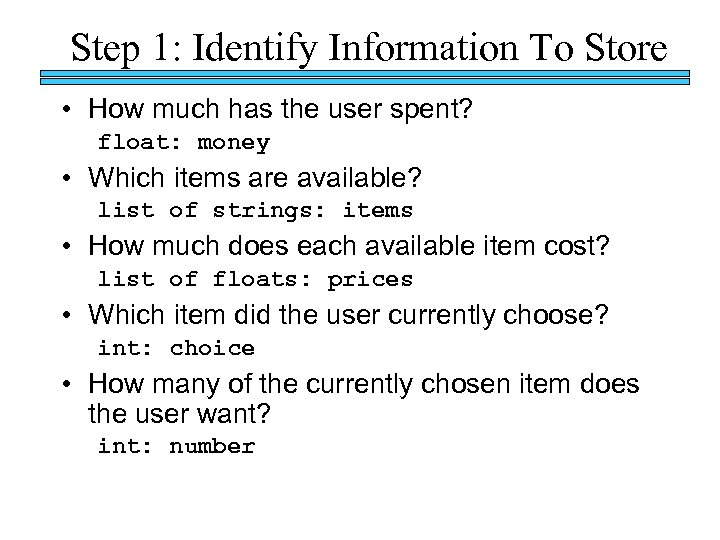Step 1: Identify Information To Store • How much has the user spent? float: money • Which items are available? list of strings: items • How much does each available item cost? list of floats: prices • Which item did the user currently choose? int: choice • How many of the currently chosen item does the user want? int: number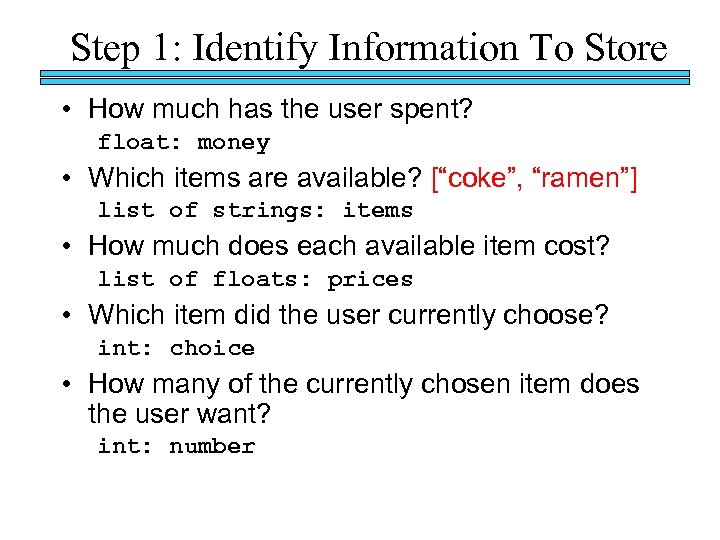Step 1: Identify Information To Store • How much has the user spent? float: money • Which items are available? [“coke”, “ramen”] list of strings: items • How much does each available item cost? list of floats: prices • Which item did the user currently choose? int: choice • How many of the currently chosen item does the user want? int: number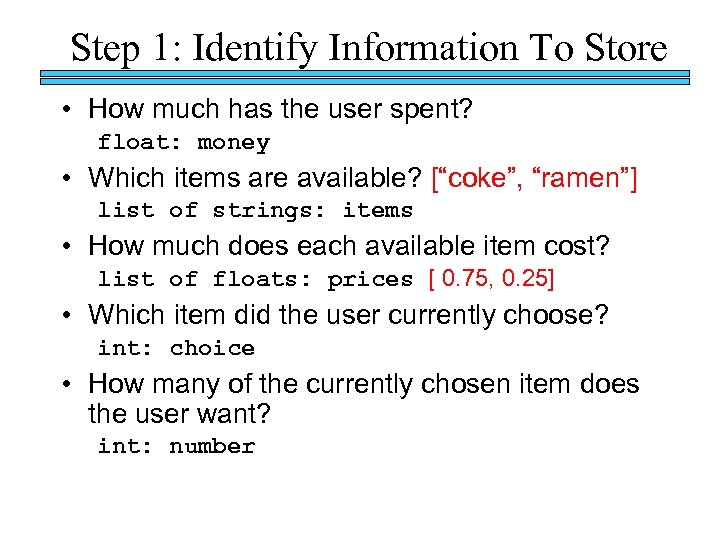Step 1: Identify Information To Store • How much has the user spent? float: money • Which items are available? [“coke”, “ramen”] list of strings: items • How much does each available item cost? list of floats: prices [ 0. 75, 0. 25] • Which item did the user currently choose? int: choice • How many of the currently chosen item does the user want? int: number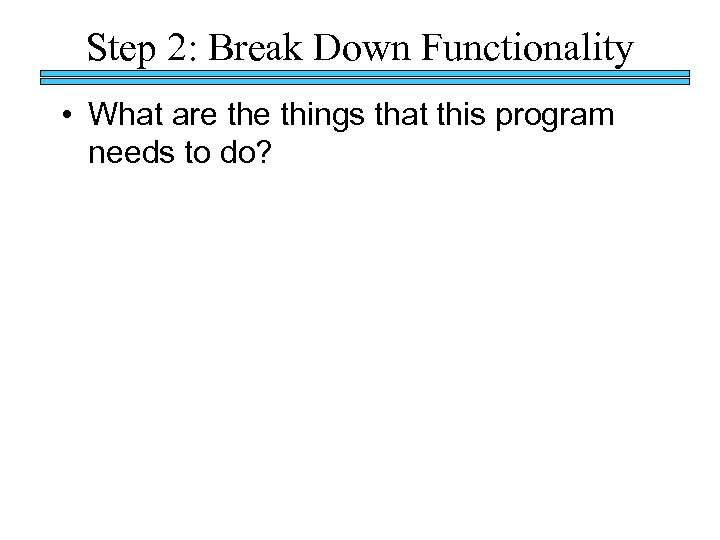Step 2: Break Down Functionality • What are things that this program needs to do?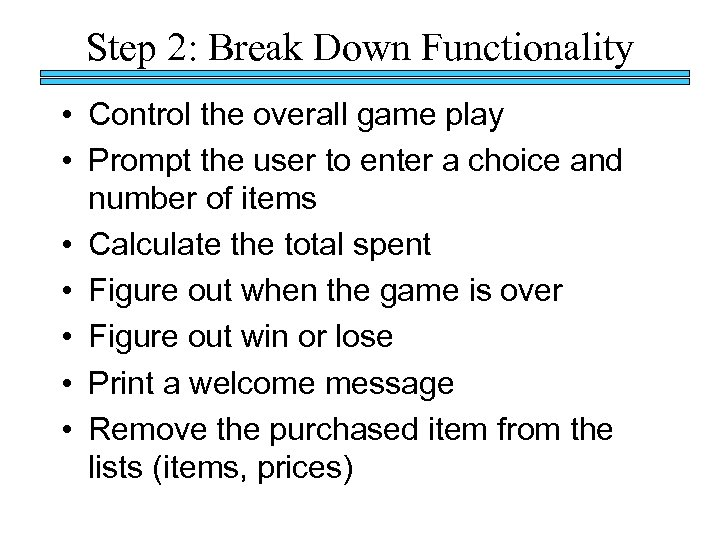Step 2: Break Down Functionality • Control the overall game play • Prompt the user to enter a choice and number of items • Calculate the total spent • Figure out when the game is over • Figure out win or lose • Print a welcome message • Remove the purchased item from the lists (items, prices)

>> 0 : 1 : 2 : items =" src="https://present5.com/presentation/4143f71dee2f0ac09b978887135c38cb/image-41.jpg" alt=""Quiz" part 1: Print Items >>> 0 : 1 : 2 : items =" /> "Quiz" part 1: Print Items >>> 0 : 1 : 2 : items = [“coke”, “pepsi”, “sprite”] print. Items(items) coke pepsi sprite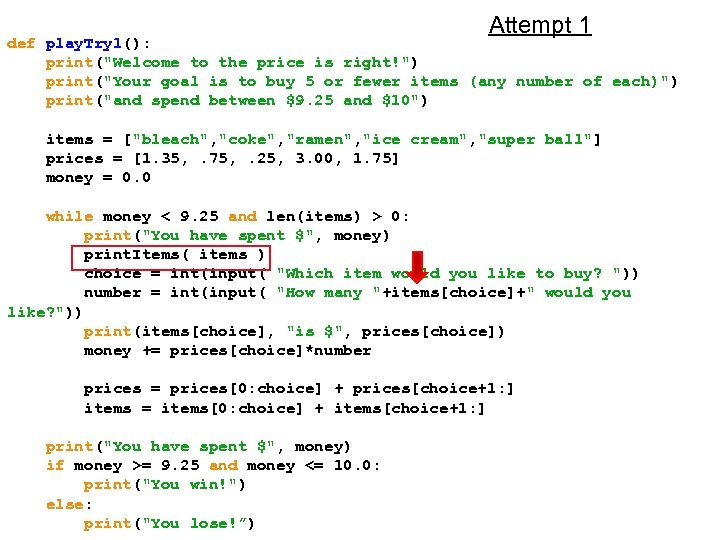Attempt 1 def play. Try 1(): print("Welcome to the price is right!") print("Your goal is to buy 5 or fewer items (any number of each)") print("and spend between \$9. 25 and \$10") items = ["bleach", "coke", "ramen", "ice cream", "super ball"] prices = [1. 35, . 75, . 25, 3. 00, 1. 75] money = 0. 0 while money < 9. 25 and len(items) > 0: print("You have spent \$", money) print. Items( items ) choice = int(input( "Which item would you like to buy? ")) number = int(input( "How many "+items[choice]+" would you like? ")) print(items[choice], "is \$", prices[choice]) money += prices[choice]*number prices = prices[0: choice] + prices[choice+1: ] items = items[0: choice] + items[choice+1: ] print("You have spent \$", money) if money >= 9. 25 and money <= 10. 0: print("You win!") else: print("You lose!”)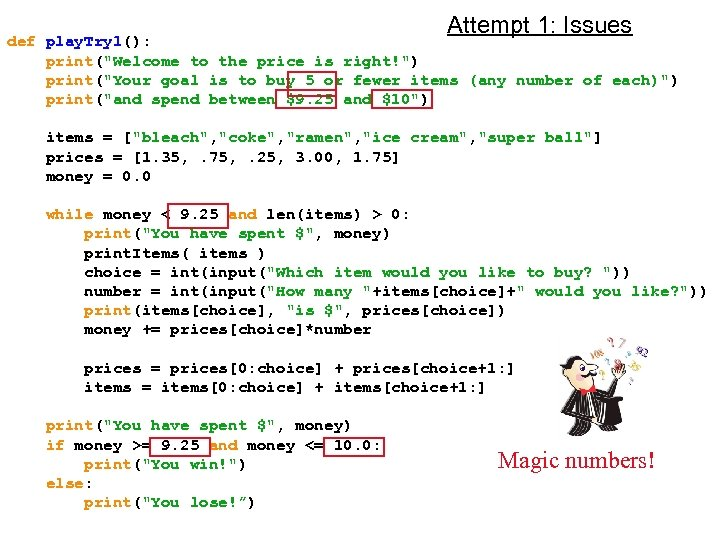Attempt 1: Issues def play. Try 1(): print("Welcome to the price is right!") print("Your goal is to buy 5 or fewer items (any number of each)") print("and spend between \$9. 25 and \$10") items = ["bleach", "coke", "ramen", "ice cream", "super ball"] prices = [1. 35, . 75, . 25, 3. 00, 1. 75] money = 0. 0 while money < 9. 25 and len(items) > 0: print("You have spent \$", money) print. Items( items ) choice = int(input("Which item would you like to buy? ")) number = int(input("How many "+items[choice]+" would you like? ")) print(items[choice], "is \$", prices[choice]) money += prices[choice]*number prices = prices[0: choice] + prices[choice+1: ] items = items[0: choice] + items[choice+1: ] print("You have spent \$", money) if money >= 9. 25 and money <= 10. 0: print("You win!") else: print("You lose!”) Magic numbers!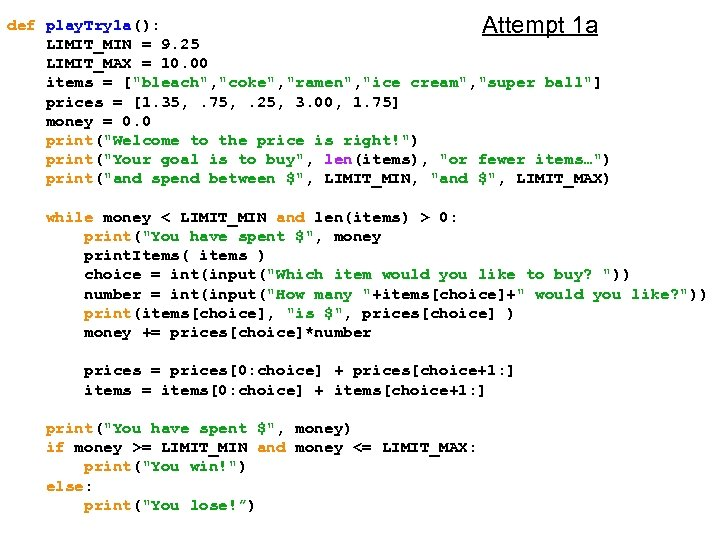def play. Try 1 a(): Attempt 1 a LIMIT_MIN = 9. 25 LIMIT_MAX = 10. 00 items = ["bleach", "coke", "ramen", "ice cream", "super ball"] prices = [1. 35, . 75, . 25, 3. 00, 1. 75] money = 0. 0 print("Welcome to the price is right!") print("Your goal is to buy", len(items), "or fewer items…") print("and spend between \$", LIMIT_MIN, "and \$", LIMIT_MAX) while money < LIMIT_MIN and len(items) > 0: print("You have spent \$", money print. Items( items ) choice = int(input("Which item would you like to buy? ")) number = int(input("How many "+items[choice]+" would you like? ")) print(items[choice], "is \$", prices[choice] ) money += prices[choice]*number prices = prices[0: choice] + prices[choice+1: ] items = items[0: choice] + items[choice+1: ] print("You have spent \$", money) if money >= LIMIT_MIN and money <= LIMIT_MAX: print("You win!") else: print("You lose!”)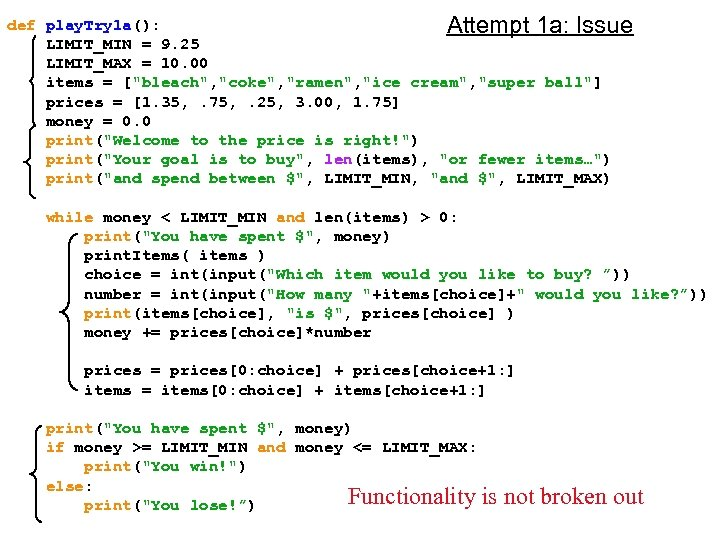def play. Try 1 a(): Attempt 1 a: Issue LIMIT_MIN = 9. 25 LIMIT_MAX = 10. 00 items = ["bleach", "coke", "ramen", "ice cream", "super ball"] prices = [1. 35, . 75, . 25, 3. 00, 1. 75] money = 0. 0 print("Welcome to the price is right!") print("Your goal is to buy", len(items), "or fewer items…") print("and spend between \$", LIMIT_MIN, "and \$", LIMIT_MAX) while money < LIMIT_MIN and len(items) > 0: print("You have spent \$", money) print. Items( items ) choice = int(input("Which item would you like to buy? ”)) number = int(input("How many "+items[choice]+" would you like? ”)) print(items[choice], "is \$", prices[choice] ) money += prices[choice]*number prices = prices[0: choice] + prices[choice+1: ] items = items[0: choice] + items[choice+1: ] print("You have spent \$", money) if money >= LIMIT_MIN and money <= LIMIT_MAX: print("You win!") else: Functionality is print("You lose!”) not broken out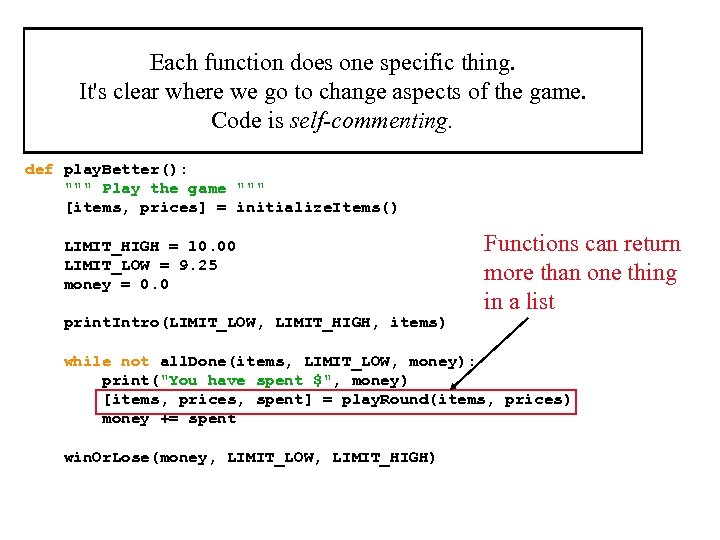Each function does one specific thing. It's clear where we go to change aspects of the game. Code is self-commenting. def play. Better(): """ Play the game """ [items, prices] = initialize. Items() LIMIT_HIGH = 10. 00 LIMIT_LOW = 9. 25 money = 0. 0 print. Intro(LIMIT_LOW, LIMIT_HIGH, items) Functions can return more than one thing in a list while not all. Done(items, LIMIT_LOW, money): print("You have spent \$", money) [items, prices, spent] = play. Round(items, prices) money += spent win. Or. Lose(money, LIMIT_LOW, LIMIT_HIGH)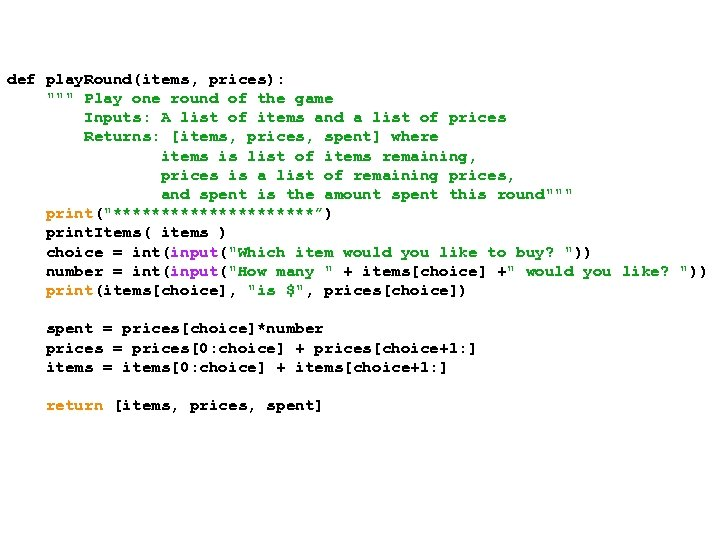def play. Round(items, prices): """ Play one round of the game Inputs: A list of items and a list of prices Returns: [items, prices, spent] where items is list of items remaining, prices is a list of remaining prices, and spent is the amount spent this round""" print("***********”) print. Items( items ) choice = int(input("Which item would you like to buy? ")) number = int(input("How many " + items[choice] +" would you like? ")) print(items[choice], "is \$", prices[choice]) spent = prices[choice]*number prices = prices[0: choice] + prices[choice+1: ] items = items[0: choice] + items[choice+1: ] return [items, prices, spent]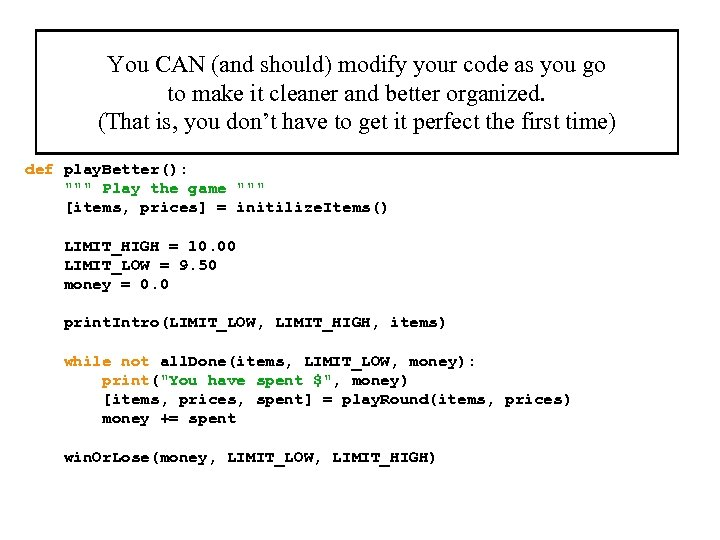You CAN (and should) modify your code as you go to make it cleaner and better organized. (That is, you don’t have to get it perfect the first time) def play. Better(): """ Play the game """ [items, prices] = initilize. Items() LIMIT_HIGH = 10. 00 LIMIT_LOW = 9. 50 money = 0. 0 print. Intro(LIMIT_LOW, LIMIT_HIGH, items) while not all. Done(items, LIMIT_LOW, money): print("You have spent \$", money) [items, prices, spent] = play. Round(items, prices) money += spent win. Or. Lose(money, LIMIT_LOW, LIMIT_HIGH)

>>" src="https://present5.com/presentation/4143f71dee2f0ac09b978887135c38cb/image-49.jpg" alt="Name(s): ________________ "Quiz" Print a list of strings with the specified format Example: >>>" /> Name(s): ________________ "Quiz" Print a list of strings with the specified format Example: >>> 0 : 1 : 2 : items = [“coke”, “pepsi”, “sprite”] print. Items(items) coke pepsi sprite You need determine each element's index… def print. Items( items ): Return the min difference between any 2 elements in L Example: >>> diff([7, 0, 6, 4]) 1 Only consider unsigned differences. You can assume at least 2 elements in the list Hint: use a NESTED loop def diff( L ):

>> 0 : 1 : 2 : items =" src="https://present5.com/presentation/4143f71dee2f0ac09b978887135c38cb/image-50.jpg" alt=""Quiz" part 1: Print Items >>> 0 : 1 : 2 : items =" /> "Quiz" part 1: Print Items >>> 0 : 1 : 2 : items = [“coke”, “pepsi”, “sprite”] print. Items(items) coke pepsi sprite

>> 0 : 1 : 2 : items =" src="https://present5.com/presentation/4143f71dee2f0ac09b978887135c38cb/image-51.jpg" alt=""Quiz" part 1: Print Items >>> 0 : 1 : 2 : items =" /> "Quiz" part 1: Print Items >>> 0 : 1 : 2 : items = [“coke”, “pepsi”, “sprite”] print. Items(items) coke pepsi sprite def print. Items(items): for x in range(len(items)): print(x, ”: ”, items[x])

>> items = [“coke”, “pepsi”, “sprite”] >>> print. Items(items)" src="https://present5.com/presentation/4143f71dee2f0ac09b978887135c38cb/image-52.jpg" alt=""Quiz" part 1: Print Items >>> items = [“coke”, “pepsi”, “sprite”] >>> print. Items(items)" /> "Quiz" part 1: Print Items >>> items = [“coke”, “pepsi”, “sprite”] >>> print. Items(items) 0: coke 1: pepsi 2: sprite def print. Items(items): for x in range(len(items)): print(str(x)+”: ”+items[x])

>> diff([7, 0, 6, 4]) 1 Only consider unsigned differences." src="https://present5.com/presentation/4143f71dee2f0ac09b978887135c38cb/image-53.jpg" alt=""Quiz" Part 2 Example: >>> diff([7, 0, 6, 4]) 1 Only consider unsigned differences." /> "Quiz" Part 2 Example: >>> diff([7, 0, 6, 4]) 1 Only consider unsigned differences. You can assume at least 2 elements in the list Hint: use a NESTED loop def diff( L ): Return the min difference between any 2 elements in L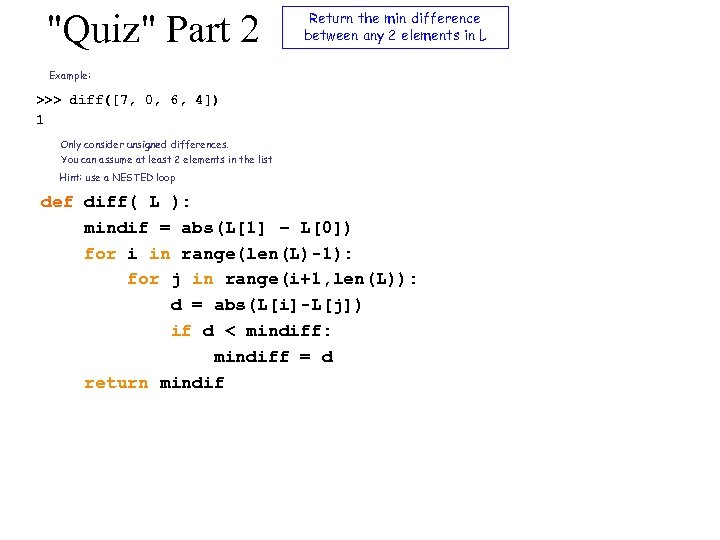"Quiz" Part 2 Return the min difference between any 2 elements in L Example: >>> diff([7, 0, 6, 4]) 1 Only consider unsigned differences. You can assume at least 2 elements in the list Hint: use a NESTED loop def diff( L ): mindif = abs(L – L) for i in range(len(L)-1): for j in range(i+1, len(L)): d = abs(L[i]-L[j]) if d < mindiff: mindiff = d return mindif